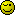# Javascript Date issues

Okay so I know I’m doing something fundamentally wrong here, but I can’t figure it out. Basically I am taking the time of a tweet format:

Wed Nov 18 18:54:12 +0000 2009

then breaking it apart into then converting it into numbers so I can put it into a date object like this:

var t=new Date(year, mon, day, hour, min, sec);

Then since twitter stores its tweet times in GMT I take off the t.getTimezoneOffset() and then subtract it from the current time.

var diff=new Date().getTime-(t.getTime()-t.getTimezoneOffset());

However this sometimes works, and sometimes gets completely wrong answers. I’m not quite sure what I’m doing wrong here. Anyway here is my code:

``````	var hold=new Date().getTime();

var t=new Date(year, mon, day, hour, min, sec);
var save=t.getTime()-(t.getTimezoneOffset()*60000);
var d=hold-save;

d=Math.floor(d/1000);

var formatTime='';
if(d<60)
formatTime=d+' seconds ago';
else if(d<3600)
formatTime=(Math.floor(d/60))+' minutes ago';
else if(d<86400)
formatTime=(Math.floor(d/3600))+' hours ago';
else
formatTime=(Math.floor(d/86400))+' days ago';

``````

Thanks.

I suspect that you may want to take into account the local timezone offset when adjusting the hours.
getTimezoneOffset

That way you can adjust the hours until you can correctly set the datetime according to either the clients local timezone.

Or, if you don’t want to do that, you can set the datetime according to UTC with setUTCHours and such like.

I thought I did, “t.getTimezoneOffset()”

Unless you think I should apply the offset before I even create the date. That seems like more work than is necessary.

The less work is to use the setUTC… methods to set the date on the local machine.

That way you only have to remove the offset from the date, and by setting the UTC time the computer will automatically work out its own local time.

Great I’ll try it out to see if it works. Just to verify is this the idea you are talking about:

``````
//I'll change this part to be dynamic based on the actual offset but for demonstration 8 works
var hold=new Date().setUTCHours(8)
var t=new Date(year, mon, day, hour, min, sec);
var difference=hold.getTime()-t.getTime();

//Proceed to actually format the difference into seconds, minutes, etc.

``````

Okay so very confused I realized that setUTCHours actually sets it to that value, so I changed it to represent that, but it still didn’t work.

``````
var t=new Date(year, mon, day, hour, min, sec);
t.setUTCHours(hour);

``````

So then I just tried taking 8 hours off the hour value and subtracting a day if it was less than 0 (I know it’s sloppy but I’m desperate)

``````
hour-=8;
if(day<0)
{
hour=24-hour;
day--;
}
var t=new Date(year, mon, day, hour, min, sec);

``````

That didn’t work either. I’m seriously at such a loss here, I still don’t understand why my original logic of just subtracting the timezoneOffset from the getTime value didn’t work. I mean isn’t that all a timezone is x hours in the past or future from your local time?

The stranger part is that only some times are incorrect for instance it’ll be showing all the times correctly and then completely start doing the times wrong.

Here’s a table of what my website says compared to what it should be

3 hours------------3 hours
3 hours------------3 hours
12 hours----------3 hours

This is another approach, relying on the format of the time string.

``````function getTweetTime(ts){
var D, tz, d= ts.split(/ +/),
h= d.splice(3, 2);
d= d+' '+d+','+d+' '+h;

D= new Date(Date.parse(d));
tz= D.getTimezoneOffset();
if(tz) D.setUTCMinutes(D.getUTCMinutes()+(-tz));
return D;
}
``````

// test case:
var time= getTweetTime(‘Wed Nov 18 18:54:12 +0000 2009’);

alert(‘When it is ‘+time.toUTCString()+’ in London, it is ‘+
time.toLocaleString()+’ here.’)

/*
getTweetTime(ts){
Split the string on spaces, and splice out the time data:

``````var D, tz, d= ts.split(/ +/),
h= d.splice(3, 2);

This gives you two arrays:

[B]d= [Wed, Nov, 18, 2009] and h= [18: 54: 12, +0000][/B]

It would make no difference if it were[Wed, 18, Nov],
they both parse correctly.
Now you can put together a string that can be parsed to local time:

d= d+' '+d+','+d+' '+h;
[B]** d now ='Nov 18,2009 18:54:12' **[/B]
var D= new Date(Date.parse(d));

If the time stamp is always set to UTC you can ignore the '+0000'
and set UTCMinutes by the local time zone offset for that date ([B]not today's date![/B]).
The offset is in + or - minutes in javascript, and is added
to the local time if less than 0, subtracted if greater.
Using -tz saves figuring.

( If the the time stamp could be  greater or less than +0000,
``````

// tz= D.getTimezoneOffset()-(parseInt(h)|| 0)``````The function returns a date with the correct local time.

tz= D.getTimezoneOffset()-(parseInt(h)|| 0);
if(tz) D.setUTCMinutes(D.getUTCMinutes()+(-tz));
return D;
``````

}
*/

Alright thanks for the descriptive response. I’ll try that out now and report back.

That did work, but one last thing. How would I go subtracting that value from the current time so I can return the time to the user in the form of x seconds/minutes/etc ago

I tried just new Date.getTime()-getTimeTweets(value).getTime() but that din’t work.

Nevermind, I forgot to divide the difference by a 1000. Anyway seems to work perfectly. Here’s the code that got it:

``````
var d=new Date().getTime();
var holdme=getTweetTime(time);
var diff=d-holdme.getTime();
diff=Math.floor(diff/1000);
if(diff<60)
formatTime=diff+' seconds ago';
else if(diff<3600)
formatTime=(Math.floor(diff/60))+' minutes ago';
else if(diff<86400)
formatTime=(Math.floor(diff/3600))+' hours ago';
else
formatTime=(Math.floor(diff/86400))+' days ago';
var save=holdme.getTime();

``````

Thanks Everyone (<3 Sitepoint)! But just one last question. Why doesn’t taking the timezone offset value from the getTime() value work?

You can subtract the dates without first getting the time-
var holdme=getTweetTime(time);
var diff=new Date()-holdme;

Both dates will be converted to UTC milliseconds, with no time zone (UTC +0).
The return in either case is an integer, not a date object.

Why thank you.